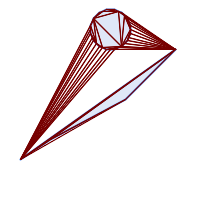## Name

ST_ConstrainedDelaunayTriangles — Return a constrained Delaunay triangulation around the given input geometry.

## Synopsis

`geometry ST_ConstrainedDelaunayTriangles(`geometry g1`)`;

## Description

Return a Constrained Delaunay triangulation around the vertices of the input geometry. Output is a TIN.This method needs SFCGAL backend.

Availability: 3.0.0This function supports 3d and will not drop the z-index.

## ExamplesST_ConstrainedDelaunayTriangles of 2 polygons ```select ST_ConstrainedDelaunayTriangles( ST_Union( 'POLYGON((175 150, 20 40, 50 60, 125 100, 175 150))'::geometry, ST_Buffer('POINT(110 170)'::geometry, 20) ) ); ```ST_DelaunayTriangles of 2 polygons. Triangle edges cross polygon boundaries. ```select ST_DelaunayTriangles( ST_Union( 'POLYGON((175 150, 20 40, 50 60, 125 100, 175 150))'::geometry, ST_Buffer('POINT(110 170)'::geometry, 20) ) ); ```